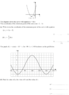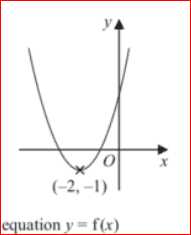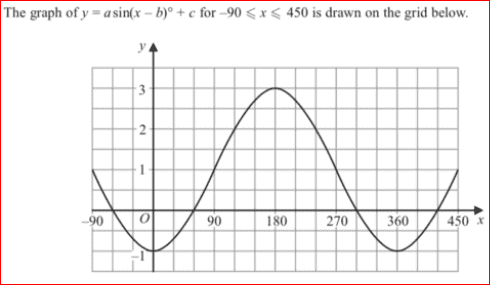# Minimum point of a curve

Natasha1
<Moderator's note: Member has been informed to separate different question into different threads in the future.>

1. Homework Statement

The diagram shows the curve with equation y = f (x)
The coordinates of the minimum point of the curve are (–2, –1)

(a) Write down the coordinates of the minimum point of the curve with equation

(i) y=f(x–5)
(ii) y = 0.5 f ( x )

The graph of y=asin(x–b)+c (please see graph on picture)
b) Find the value of a, the value of b and the value of c.

## The Attempt at a Solution

(a) Write down the coordinates of the minimum point of the curve with equation

(i) y=f(x–5) Does the -5 move the graph 5 units to the right? Why is this? So the coordinates are (-7, -1)
(ii) y = 0.5 f(x) Does the times 0.5 widen the curve and has no impact on the minimum point so would stay (-2, 1)

b) Find the value of a, the value of b and the value of c.
Is a the magnitude? so a = 1
Is b the phase shift so -b shifts the graph by b units to the right so b = 360
Is c the vertical shift which is c = -1

#### Attachments

•Screenshot 2019-03-08 at 19.19.51.png
16.4 KB · Views: 945
Last edited by a moderator:

Staff Emeritus
Homework Helper
Gold Member

## Homework Statement

The diagram shows the curve with equation y = f (x)
The coordinates of the minimum point of the curve are (–2, –1)

(a) Write down the coordinates of the minimum point of the curve with equation

(i) y=f(x–5)
(ii) y = 0.5 f ( x )

The graph of y=asin(x–b)+c (please see graph on picture)
b) Find the value of a, the value of b and the value of c.

## The Attempt at a Solution

(a) Write down the coordinates of the minimum point of the curve with equation

(i) y=f(x–5) Does the -5 move the graph 5 units to the right? Why is this? So the coordinates are (-7, -1)
(ii) y = 0.5 f(x) Does the times 0.5 widen the curve and has no impact on the minimum point so would stay (-2, 1)

b) Find the value of a, the value of b and the value of c.
Is a the magnitude? so a = 1
Is b the phase shift so -b shifts the graph by b units to the right so b = 360
Is c the vertical shift which is c = -1

Even though these are shown as parts (a) and (b) on the image, the parts have almost nothing in common and it would make sense to post each part in a separate thread.
Graph for part (a):Graph for part (b):#### Attachments

•Natasha1
Staff Emeritus
Homework Helper
Gold Member
Hint for Part (a):
You should know the value of ƒ(−2). Right?

So, what value must x have in order for ƒ(x − 5) to give the value of ƒ(−2) ?

Natasha1
Even though these are shown as parts (a) and (b) on the image, the parts have almost nothing in common and it would make sense to post each part in a separate thread.
Graph for part (a):
View attachment 239951

Graph for part (b):
View attachment 239952
Hint for Part (a):
You should know the value of ƒ(−2). Right?

So, what value must x have in order for ƒ(x − 5) to give the value of ƒ(−2) ?

ƒ(−2) = -1
So, what value must x have in order for ƒ(x − 5) to give the value of ƒ(−2) ? I don't know ƒ(x − 5) = -1

Staff Emeritus
Homework Helper
Gold Member
ƒ(−2) = -1
So, what value must x have in order for ƒ(x − 5) to give the value of ƒ(−2) ? I don't know ƒ(x − 5) = -1
Then, what value must x have so that (x − 5) = −2 ?

Natasha1
x = 3

Natasha1
x = 3

Staff Emeritus
Homework Helper
Gold Member
x = 3
Right.
So, if you are graphing ƒ(x−5), then when x = 3, y = ƒ(3 − 5) = ƒ(−2) = −1 , does it not?

Thus, the graph of y = ƒ(x−5) is just like the graph of y = ƒ(x), except that
the graph, y = ƒ(x−5) is shifted 5 units to the right as compared to the graph of y = ƒ(x) .

•Natasha1
Natasha1
Thanks SammyS but what about the rest of my work?

Staff Emeritus
Homework Helper
Gold Member
Thanks SammyS but what about the rest of my work?

(a) : (i)
It is true that the graph, y = ƒ(x − 5), is obtained from the graph, y = ƒ(x), by shifting the latter by 5 units to the right, which is what you said, but then your answer of (−7, −1) for the coordinates of the vertex (a minimum) is incorrect. You need to shift the graph, not the coordinate system. Also, I gave you some idea of how to think of this as a shift.

(a) : (ii)
While it is true that multiplying ƒ(x) by 0.5 gives a graph that appears to be widened (at least for this function), what multiplying by 0.5 actually does is to "shrink" the graph vertically. This is because for any particular x value, the y value for y = 0.5ƒ(x) is one half of the y value for y = ƒ(x) .

Natasha1

(i) y=f(x–5) Does the -5 move the graph 5 units to the right? Why is this? So the coordinates are (3, -1)
(ii) y = 0.5 f(x) Does the times 0.5 widen the curve and has no impact on the minimum point so would stay (-2, -1)

Natasha1